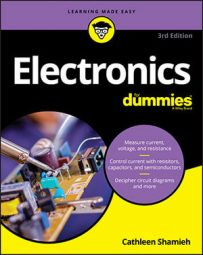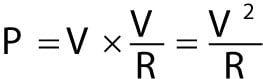##### Electronics For DummiesA scientist hard at work in the early 1800s was the energetic James Prescott Joule. Joule is responsible for coming up with the equation that gives you power values; it's known as Joule's Law:

P = V x I

This equation states that the power (in watts) equals the voltage (in volts) across a component times the current (in amps) passing through that component. The nice thing about this equation is that it applies to every electronic component, whether it's a resistor, a light bulb, a capacitor, or something else. It tells you the rate at which electrical energy is consumed by the component — what that power is.

## Using Joule's Law to choose components

You've already seen how to use Joule's Law to ensure that a resistor is big enough to resist a meltdown in a circuit, but you should know that this equation comes in handy also when you're selecting other electronic parts.

Lamps, diodes, and other components also come with maximum power ratings. If you expect them to perform at power levels higher than their ratings, you're going to be disappointed when too much power makes them pop and fizzle. When you select the part, you should consider the maximum possible power the part will need to handle in the circuit. You do this by determining the maximum current you'll be passing through the part and the voltage across the part, and then multiplying those quantities together. Then you choose a part with a power rating that exceeds that estimated maximum power.

## Joule and Ohm: perfect together

You can get creative and combine Joule's Law and Ohm's Law to derive more useful equations to help you calculate power for resistive components in circuits. For instance, if you substitute I x R for V in Joule's Law, you get:

P = (I x R) x I = I2R

That gives you a way to calculate power if you know the current and resistance but not the voltage. Similarly, you can substitute V/R for I in Joule's Law to getUsing that formula, you can calculate power if you know the voltage and the resistance but not the current.

Joule's Law and Ohm's Law are used in combination so often that Georg Ohm sometimes gets the credit for both laws!